2022: SklogWiki celebrates 15 years on-line

# Grand canonical Monte Carlo

Grand-canonical ensemble Monte Carlo (GCEMC or GCMC) is a very versatile and powerful Monte Carlo technique that explicitly accounts for density fluctuations at fixed volume and temperature. This is achieved by means of trial insertion and deletion of molecules. Although this feature has made it the preferred choice for the study of interfacial phenomena, in the last decade grand-canonical ensemble simulations have also found widespread applications in the study of bulk properties. Such applications had been hitherto limited by the very low particle insertion and deletion probabilities, but the development of the configurational bias grand canonical technique has very much improved the situation.

## Theoretical basis

In the grand canonical ensemble, one first chooses randomly whether a trial particle insertion or deletion is attempted. If insertion is chosen, a particle is placed with uniform probability density inside the system. If deletion is chosen, then one deletes one out of$N$ particles randomly. The trial move is then accepted or rejected according to the usual Monte Carlo lottery. As usual, a trial move from an original state (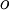$o$) to a new state (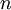$n$) is accepted with probability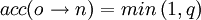$acc(o \rightarrow n) = min \left (1, q \right )$

where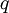$q$ is given by: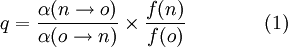$q = \frac{ \alpha(n \rightarrow o)}{\alpha(o \rightarrow n)} \times \frac{f(n)}{f(o)} \qquad\qquad\text{(1)}$

Here,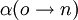$\alpha(o \rightarrow n)$ is the probability density of attempting trial move from state$o$ to state$n$ (also known as underlying probability), while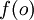$f(o)$ is the probability density of state$o$.

As noted by Norman and Filinov , evaluation of the proper acceptance rules requires very careful interpretation of the (classical) grand canonical probability density: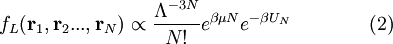$f_L({\mathbf r}_1,{\mathbf r}_2 ..., {\mathbf r}_N) \propto \frac{\Lambda^{-3N}}{N!} e^{\beta \mu N} e^{-\beta U_N}\qquad\qquad\text{(2)}$

where$N$ is the total number of particles,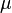$\mu$ is the chemical potential,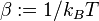$\beta := 1/k_B T$ and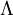$\Lambda$ is the de Broglie thermal wavelength.

The sub-index L makes emphasis on a particular definition of microstate in which particles are assumed to be distinguishable. Thus,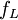$f_L$ should be interpreted as the probability density of a classical state with labelled particles, having labelled particle 1 in position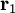${\mathbf r}_1$, labelled particle 2 in position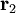${\mathbf r}_2$ and so on. Since labelling of the particles has no physical significance whatsoever, there are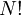$N!$ identical states which result from permutation of the labels (this explains the$N!$ term in the denominator). Hence, the probability of the significant microstate of undistinguishable particles, i.e., one with$N$ particles at positions${\mathbf r}_1$,${\mathbf r}_2$, etc., irrespective of the labels, will be given by: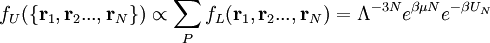$f_U( \{{\mathbf r}_1,{\mathbf r}_2 ..., {\mathbf r}_N \} ) \propto \sum_P f_L({\mathbf r}_1,{\mathbf r}_2 ..., {\mathbf r}_N) = \Lambda^{-3N} e^{\beta \mu N} e^{-\beta U_N}$

where the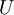$U$ subindex stands for unlabelled, the coordinates in parenthesis indicate that the positions are not attributed to any particular choice of labelling and the sum runs over all possible particle label permutations.

Upon trial insertion of an extra particle, one obtains: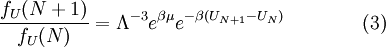$\frac{f_U(N+1)}{f_U(N)} = \Lambda^{-3} e^{\beta \mu } e^{-\beta ( U_{N+1} - U_N )}\qquad\qquad\text{(3)}$

The probability density of attempting an insertion is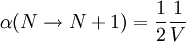$\alpha( N \rightarrow N+1 ) = \frac{1}{2} \frac{1}{V}$. The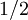$1/2$ factor accounts for the probability of attempting an insertion (from the choice of insertion or deletion). The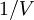$1/V$ factor results from placing the particle with uniform probability anywhere inside the simulation box. The reverse attempt (moving from state of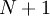$N+1$ particles to the original$N$ particle state) is chosen with probability: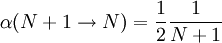$\alpha( N+1 \rightarrow N ) = \frac{1}{2} \frac{1}{N+1}$

where the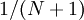$1/(N+1)$ factor results from random removal of one among$N+1$ particles. Therefore, the ratio of underlying probabilities is: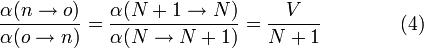$\frac{\alpha(n\rightarrow o)}{\alpha(o\rightarrow n)} = \frac{\alpha( N+1 \rightarrow N )}{\alpha( N \rightarrow N+1)} = \frac{V}{N+1} \qquad\qquad\text{(4)}$

Substitution of Eq.(3) and Eq.(4) into Eq.(1) yields the acceptance probability for attempted insertions: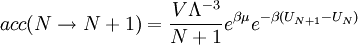$acc(N \rightarrow N+1) = \frac{V \Lambda^{-3} }{N+1} e^{\beta \mu } e^{-\beta ( U_{N+1} - U_N )}$

For the inverse deletion process, similar arguments yield: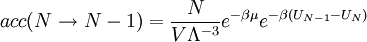$acc(N \rightarrow N-1) = \frac{N}{V \Lambda^{-3} } e^{-\beta \mu } e^{-\beta ( U_{N-1} - U_N )}$

Alternatively, one could derive the acceptance rules by considering the probability density of labelled states, Eq.(2) but taking into account that there are then$N+1$ labelled microstates leading to the original$N$ particle labelled state upon deletion (one for each possible label permutation of the deleted particle).

The same acceptance rules are obtained in reference books. Usually the problem of proper counting of states is circumvented by ignoring the labelling problem and assuming that the underlying probabilities for insertion and removal are equal.